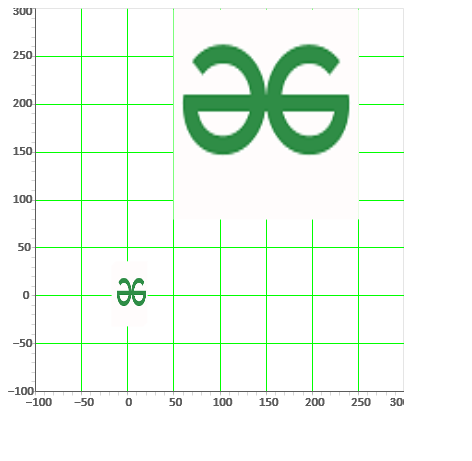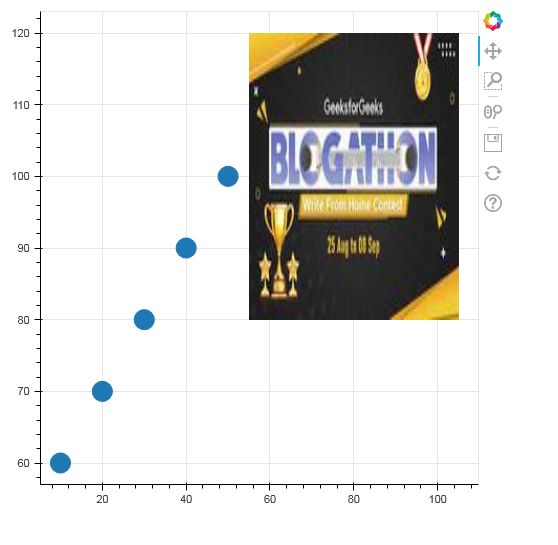Open in App
Not now

# How To Work With Images in Bokeh

• Difficulty Level : Hard
• Last Updated : 14 Sep, 2021

In this article, we are going to see how to work with images in Bokeh.

For this, we will use the ImageURL Glyph. This glyph renders images that are loaded from the given URLs.

Syntax: ImageURL(‘url’, ‘x’, ‘y’, ‘w’, ‘h’, ‘angle’, ‘dilate’)

Arguments:

• url: The url from where the images needs to be retrieved.
• x = NumberSpec(default=field(“x”), the x-coordinates to locate the image anchors.
• y = NumberSpec(default=field(“y”),  the y-coordinates to locate the image anchors.
• w = NullDistanceSpec, the width of the plot region that the image will occupy in data space.The default value is “None“, in which case the image will be displayed at its actual image size.
• h = NullDistanceSpec, the height of the plot region that the image will occupy in data space.The default value is “None“, in which case the image will be displayed at its actual image size (regardless of the units specified here).
• angle = AngleSpec(default=0), the angles with which the images are to be rotated, as measured from the horizontal.
• global_alpha = Float(1.0), an overall opacity that each image is rendered with.
• dilate = Bool(False), Whether to always round fractional pixel locations in such a way as to make the images bigger.This setting may be useful if pixel rounding errors are causing images to have a gap between them, when they should appear flush.
• anchor = Enum(Anchor), What position of the image should be anchored at the `x`, `y` coordinates.
• retry_attempts = Int(0), number of attempts to retry loading the images from the specified URL. Default is zero.
• retry_timeout = Int(0), timeout (in ms) between retry attempts to load the image from the specified URL. Default is zero ms.

### Example 1: Importing image from URL

In this example, we are loading the image from the URL given and then rendering the image on the plot. In this example, we are loading the image from the web which can also be loaded locally. Here, we are showing two images on the plot with different parameter values (such as x,y,w,h, etc). You can observe the output to better understand.

## Python

 `# importing numpy package and other libraries ` `import` `numpy as np ` `from` `bokeh.io ``import` `curdoc, show ` `from` `bokeh.models ``import` `ColumnDataSource, Grid, ImageURL, LinearAxis, Plot, Range1d ` `from` `bokeh.plotting ``import` `figure ` ` `  `# url to load image from ` `url ``=` `"https:``/``/``media.geeksforgeeks.org``/``wp``-``content``/``\ ` `uploads``/``20210829161730``/``logo.png" ` ` `  `N ``=` `5` ` `  `# creating columndatasource ` `source ``=` `ColumnDataSource(``dict``( ` `    ``url``=``[url]``*``N, ` `    ``x1``=``np.arange(N), ` `    ``y1``=``np.arange(N), ` `    ``w1``=``[``35``]``*``N, ` `    ``h1``=``[``64``]``*``N, ` `)) ` ` `  `# creating x and y axis range ` `xdr ``=` `Range1d(start``=``-``100``, end``=``300``) ` `ydr ``=` `Range1d(start``=``-``100``, end``=``300``) ` ` `  `# creating a plot with above x and y axes range ` `plot ``=` `Plot( ` `    ``title``=``None``, x_range``=``xdr, y_range``=``ydr, ` `  ``plot_width``=``400``, plot_height``=``400``, ` `    ``min_border``=``0``, toolbar_location``=``None``) ` ` `  `# loading the image using imageUrl ` `image ``=` `ImageURL(url``=``["https:``/``/``media.geeksforgeeks.org``/``\ ` `wp``-``content``/``uploads``/``20210829161730``/``logo.png"], ` `                 ``x``=``50``, y``=``80``, w``=``200``, h``=``250``, anchor``=``"bottom_left"``) ` `image1 ``=` `ImageURL(url``=``"url"``, x``=``"x1"``, y``=``"y1"``, w``=``"w1"``, ` `                  ``h``=``"h1"``, anchor``=``"center"``) ` ` `  `# rendering the images to the plot ` `plot.add_glyph(source, image) ` `plot.add_glyph(source, image1) ` ` `  `xaxis ``=` `LinearAxis() ` `plot.add_layout(xaxis, ``'below'``) ` ` `  `yaxis ``=` `LinearAxis() ` `plot.add_layout(yaxis, ``'left'``) ` ` `  `# adding grid lines to the plot ` `plot.add_layout(Grid(dimension``=``0``, ticker``=``xaxis.ticker, ` `                     ``grid_line_color``=``'#00ff00'``)) ` ` `  `plot.add_layout(Grid(dimension``=``1``, ticker``=``yaxis.ticker, ` `                     ``grid_line_color``=``'#00ff00'``)) ` ` `  `# creates output file ` `curdoc().add_root(plot) ` `curdoc().theme ``=` `'caliber'` ` `  `# showing the plot on output file ` `show(plot) `

Output:#### Explanation:

• We are creating ColumnDataSource which provides data to the Glyphs of our plot. Bokeh has its own data structure called ColumnDataSource which can be used as an input to any Bokeh object. We are creating the data with NumPy arrays.
• Now create the x and y range using range1d which creates a range in a scalar dimension and then assign it to xdr and ydr which we will use while creating the plot.
• We then create the plot with xdr and ydr as x_range and y_range and setting the height and width of the plot as 400.
• We are then retrieving the image from the URL we declared before by setting the values of all the parameters as shown in the code. We are using two images over here by giving different values.
• Now, we render the images to the plot.
• Next, we add the grid lines to the plot using the Grid function and give it the color ‘green’.
• Then, we add the plot to the app code curdoc which represents a new document and is sent to the bokehJs to show it to the user.

### Example 2: Creating Circle glyph

In this example, we are creating a figure with a circle glyph and then simply rendering the image from the given URL using the glyph ImageURL.

## Python

 `# importing numpy package and other libraries ` `import` `numpy as np ` `from` `bokeh.io ``import` `curdoc, show ` `from` `bokeh.models ``import` `ColumnDataSource, Grid, ImageURL, LinearAxis, Plot, Range1d ` `from` `bokeh.plotting ``import` `figure ` ` `  `# create dict as basis for ColumnDataSource ` `data ``=` `{``'x1'``: [``10``, ``20``, ``30``, ``40``, ``50``], ` `        ``'y1'``: [``60``, ``70``, ``80``, ``90``, ``100``]} ` ` `  `# create ColumnDataSource based on dict ` `source ``=` `ColumnDataSource(data``=``data) ` ` `  `# creating figure ` `p ``=` `figure(plot_height``=``500``, plot_width``=``500``) ` `p.circle(x``=``"x1"``, y``=``"y1"``, size``=``20``, source``=``source) ` ` `  `# rendering the image using imageUrl ` `image ``=` `ImageURL(url``=``["https:``/``/``media.geeksforgeeks.org``/``\ ` `wp``-``content``/``uploads``/``20210902155548``/``imgurl2``-``200x112``.jpg"], ` `                 ``x``=``80``, y``=``100``, w``=``50``, h``=``40``, anchor``=``"center"``,  ` `                 ``retry_attempts``=``2``) ` ` `  `# adding the images to the plot ` `p.add_glyph(source, image) ` ` `  `# creates output file ` `curdoc().add_root(p) ` ` `  `# showing the plot on output file ` `show(p) `

Output:My Personal Notes arrow_drop_up
Related Articles